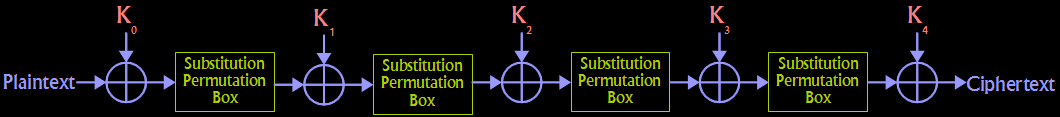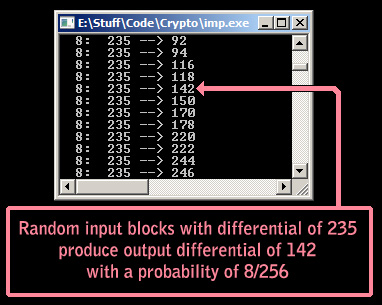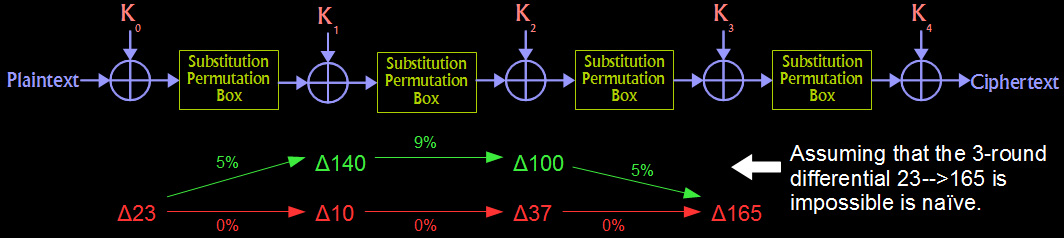# Impossible Differential Cryptanalysis

So we've fiddled with differential attacks quite a bit at this point. In this tutorial, you'll learn about a cool variant of it called impossible differential cryptanalysis (sometimes abbreviated IDC). In normal differential analysis, we try to find a differential characteristic that holds true with some high probability. If this characteristic does from the plaintext to the input to the last round, we can have a go at some straight-forward key recovery. If you need a refresher, I recommend checking out my page on the differential cryptanalysis of FEAL-4. Impossible differentials are a natural idea: instead of looking for high-probability differentials, we look for those that never happen. This give information about intermediate states in the cipher that differ from a random permutation. Exploiting these weaknesses can enable us to recover the key with less work than exhaustive search.

## Source Code

Here is the code I wrote that demonstrates the technique described on this page. Writing clear code that is easy to understand by humans is not a strong point of mine. Be sure that you have the diagrams on this page in front of you (preferably printed out) while reading the code. Enjoy!

Impossible Differentials Attack Code - [imp.c]

### The Target Cipher

The target of our attack here is our old friend from the Multi-round Differential Cryptanalysis page. For all of the details about how this cipher operates, check out that page. I will warn the reader, however, that the key recovery method described there is very different from this one. Frankly its a little bit weird because I figure it out before I understood the "standard" attack methodology. The key recovery method discussed on this page is conventional, however. In any case, check that page for details on the cipher we'll be going after like sbox/pbox tables. Also note that this version is 5 rounds instead of 4.This little toy cipher is just something I threw together a while back that is easy to analyze. It uses an 8 bit block size and a 40 bit key divided into 5 subkeys that each 8 bits in length. It consists of 5 identical rounds. Each round XORs the proper subkey and then runs the result through an "SP-Box". This function is composed of two identical 4 bit S-Boxes that are combined by an 8 bit P-Box. You'll notice that the 5th round does not included a final SP-Box. This is because the box is invertible and would provide no additional security. The attacker can simple run the ciphertext through the SP-Box backwards to reach the end state shown in the diagram above.

### Finding Differential Characteristics

The first step to this attack is to analyze the substitution-permutation box to build a differential distribution table. In other words, we want to know that if a particular differential X is fed into the SP-Box, what is the probability that it will produce an output differential Y. Do this for every possible X and every possible Y and keep a record of the results.In previous tutorials, we combed through the results of this exhaustive search for differential probabilities. Not so here! Instead, we simply store the data for use by the code later. An important note is that we want the results of all of this testing. Storing only the impossible characteristics is not the goal at this stage.

### Impossible Differentials

Now we have a giant table in memory that tells us what the probability will be for an input differential going through the SP-Box to produce a particular output differential. The next task is to programmatically go through this table and find characteristics that will NEVER hold true after 3 rounds through the cipher. Check out the cipher diagram at the top of this page; the only non-linear bits are these SP-Boxes. The XORing of the subkeys will not have an effect on the differentials as they travel.

Thus, we should start from every conceivable input and see if a chain of 3 SP-Boxes can lead to every conceivable output. If we find a particular input differential X cannot lead to a particular output differential Y, we have found an 3-round impossible differential. Remember that you can't just string together differentials (of zero probability in this case) like you can in a normal differential attack. The issue is that other paths may have been taken in the intermediate rounds and could still reconverge on your "impossible" output. The way to find out if this can happen is to check every single path. I wrote a little recursive function called isPath() that I recommend checking out in the source code for this purpose.At the end of this process of checking all of those possible paths through the cipher, you will be left with a list of 3-round differential characteristics that are impossible (there are 29 of them).

### Crossing Subkeys Off

The last part of the process is to choose one of the 3-round impossible differentials and encrypt some chosen-plaintext (use the input part of the imp. diff). Now we know for a fact that the input to the last round cannot be the output differential. We will use this fact to narrow down the keyspace for the last-round subkey until left with one candidate (the correct one!). The attacker should XOR his ciphertext pairs with a guess at the last-round subkey; try them all. Next, run those results backwards through the final SP-Box. Finally, XOR each of two parts of the pair together to get the differential. If it matches the output part of the 3-round impossible differential that we chose the plaintext with, IT IS THE WRONG SUBKEY. Cross it off your list.Experiment with how many chosen-plaintext pairs are needed per impossible differential tested. Also experiment with how many impossible differential are needed. I'm testing every 3-round differential (all 39 of them) and encrypting 100 plaintext pairs per diff. This seems to narrow the keyspace down to 1 correct subkey almost every time.